# 0.PTA得分截图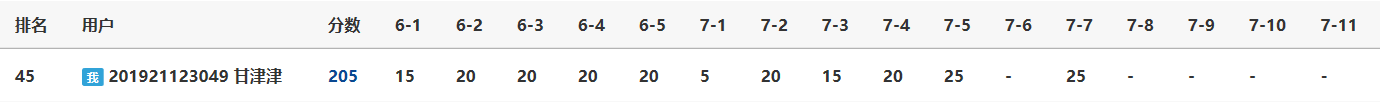# 1.本周学习总结

## 1.1 栈

### 1.1.1栈的定义及相关概念

• 栈是一种只能在一端进行插入或删除操作的线性表。

• 允许进行插入、删除操作的一端称为栈顶。

• 表的另一端称为栈底。

• 当栈中没有数据元素时，称为空栈。

• 栈的插入操作通常称为进栈或入栈，删除操作通常称为退栈或出栈。

### 1.1.2栈的存储结构

• 顺序存储，如图所示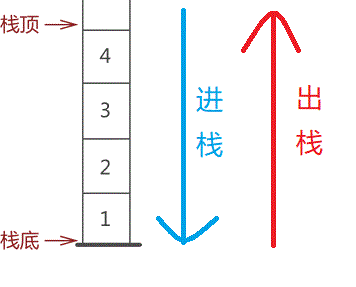• 链式存储，如图所示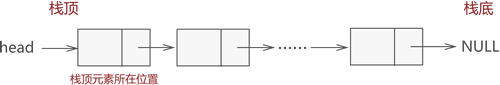### 1.1.2栈的结构体定义

typedef struct
{
int data[MAXSIZE];
int top;
}Stack;


### 1.1.3栈的初始化

SeqStack* InitStack()
{
Stack* s;
s = (Stack*)malloc(sizeof(Stack));
s->top = -1;
return s;
}


### 1.1.4入栈操作

int PushStack(Stack* s, int x)
{
if(s->top == MAXSIZE - 1)
return 0;
else{
s->top++;
s->data[s->top] = x;
return 1;
}
}

• 图解：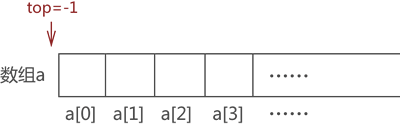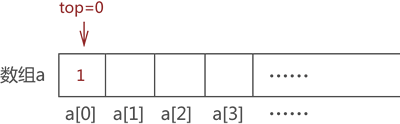### 1.1.5出栈操作

int PopStack(Stack* s, int* x)
{
if(EmptyStack(s))
return 0;            //栈空不能出栈
else
{
*x = s->data[s->top];
s->top--;
return 1;
}
}

• 图解：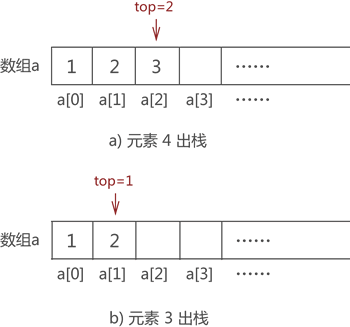### 1.1.6判断栈空操作

int EmptyStack(Stack* s)
{
if(s->top == -1)
return 1;
else
return 0;
}


### 1.1.7取栈顶元素操作

DataType GetTop(Stack* s)
{
if(EmptyStack(s))
return 0;            //栈空返回
else
return s->data[s->top];
}


### 1.1.8输出栈操作

int Print_SeqStack(SeqStack* s)
{
int i;
if(EmptyStack(s))
{
printf("空栈！\n");
return 0;            //栈空返回
}
printf("当前栈中的元素:\n");
for(i = s->top; i >= 0; i--)
printf("%d ", s->data[i]);
printf("\n");
return 0;
}


### 1.1.9链栈

• 定义：将链表的头部作为栈顶，尾部作为栈底

• 链栈的结构体定义

typedef struct node
{
int data;          /*数据域*/
struct node * next;     /*指针域*/

• 判断空栈

int StackEmpty(LinkStack *top)
{
if(!top)
return 0;
else
return 1;
}

• 入栈操作，即将数据从链表的头部插入

LinkStack *Push(LinkStack *top,int x)
{
p->data=x;         /*设置新结点的值*/
p->next=top;       /*将新元素插入栈中*/
top=p;             /*将新元素设为栈顶*/
}

• 出栈操作，即删除链表头部的首元节点

LinkStack *Pop(LinkStack *top)
{
if(!top)
{
printf("空栈！/n");
return NULL;
}
p=top;  //指向被删除的栈顶
top=top->next; //修改栈顶指针
free(p);
}

• 取栈顶元素

int GetTop(LinkStack *top)
{
if(!top)
{
printf("空栈！/n");
return 0;
}
}


### 1.2.1队列的定义及相关概念

• 队列是一种运算受限的线性表,只能选取一个端点进行插入操作，另一个端点进行删除操作。

• 进行插入(进队或入队)的一端称做队尾（rear）。

• 进行删除(出队)的一端称做队首或队头（front）。

### 1.2.2队列的存储结构

• 顺序存储，如图所示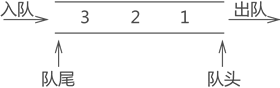• 链式存储，如图所示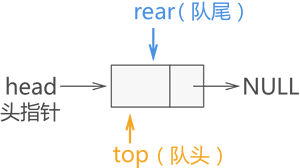### 1.2.3队列的结构体定义

typedef struct
{
int data[MaxSize]；
int front,rear；
}Queue;


### 1.2.4队列的初始化

void InitQueue(Queue *&queue)
{
q=(Queue *)malloc (sizeof(Queue));


q->front=q->rear=-1;
}

### 1.2.5入队操作

int InQueue(Queue *&q,int e)
{
if (q->rear==MaxSize-1)	        //队满
return 0;
q->rear++;
q->data[q->rear]=e;
return 1;
}


### 1.2.6出队操作

int OutQueue(Queue *&q,int &e)
{
if (q->front==q->rear)　　//队空
return 0;
q->front++;
e=q->data[q->front];
return 1;
}


### 1.2.7循环队列

• 循环队列判空的条件是front=rear。

• 循环队列判满的条件是front=（rear+1)%MaxSize。

• 循环队列结构体定义

typedef struct
{
int *base; // 初始化的动态分配存储空间
int front; // 头指针，若队列不空，指向队列头元素
int rear; // 尾指针，若队列不空，指向队列尾元素的下一个位置
}SqQueue;

• 循环队列初始化

Queue* InitQueue()
{
SqQueue *Q = (SqQueue*)malloc(sizeof(SqQueue));
Q->base = (int *)malloc(MAX_QSIZE * sizeof(int));
Q->front = Q->rear = 0;
return Q;
}

• 循环队列入队

int InQueue(Queue *Q, int e)
{
if ((Q->rear + 1) % MAX_QSIZE == Q->front) // 满队
return 0;
Q->base[Q->rear] = e;
Q->rear = (Q->rear + 1) % MAXSIZE;
return 1;
}

• 循环队列出队

int OutQueue0(Queue *Q, int &e)
{
if (Q->front == Q->rear) // 空队
return 0;
e = Q->base[Q->front];
Q->front = (Q->front + 1) % MAXSIZE;
return 1;
}


### 1.2.8链队

• 链队的结构体定义

typedef struct QNode
{
int data;
struct QNode * next;
}QNode;

• 链队的初始化

QNode * initQueue()
{
QNode * queue=(QNode*)malloc(sizeof(QNode));
queue->next=NULL;
return queue;
}

• 链队入队操作

QNode* enQueue(QNode * rear,int data)
{
QNode * temp=(QNode*)malloc(sizeof(QNode));
temp->data=data;
temp->next=NULL;
rear->next=temp;
rear=temp;
return rear;
}

• 链队出队操作

void DeQueue(QNode * top,QNode * rear)
{
if (top->next==NULL)
{
printf("队列为空");
return ;
}
QNode * p=top->next;
printf("%d",p->data);
top->next=p->next;
if (rear==p)
rear=top;
free(p);
}


# 2.PTA实验作业

## 2.1.题目1：银行业务队列简单模拟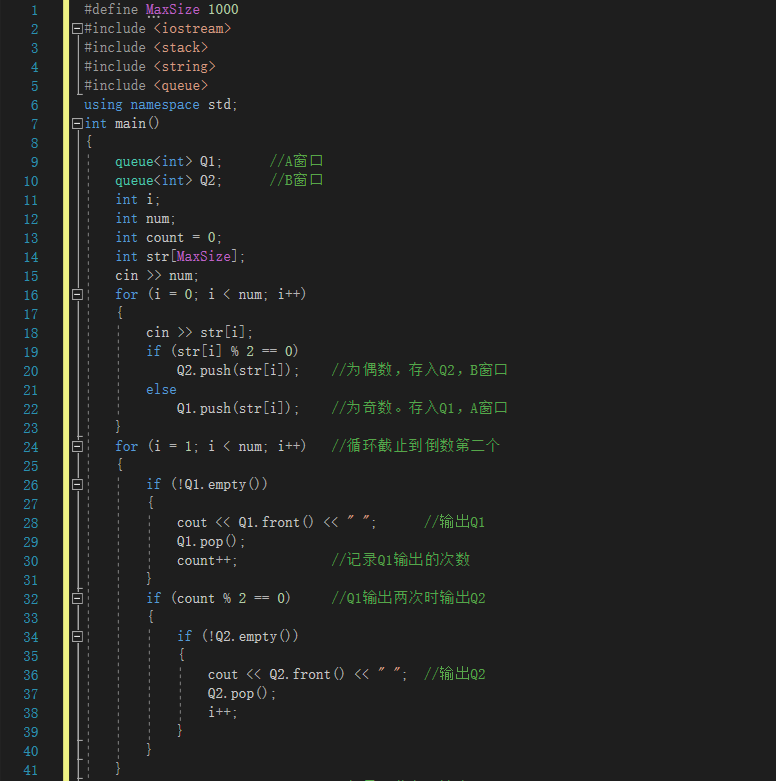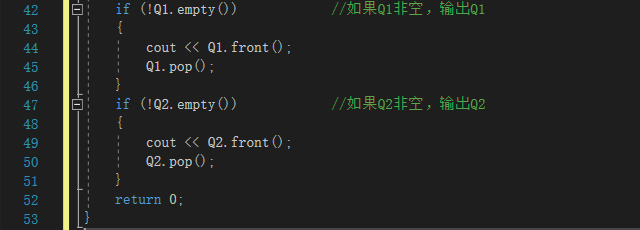## 2.1.2本题PTA提交列表说明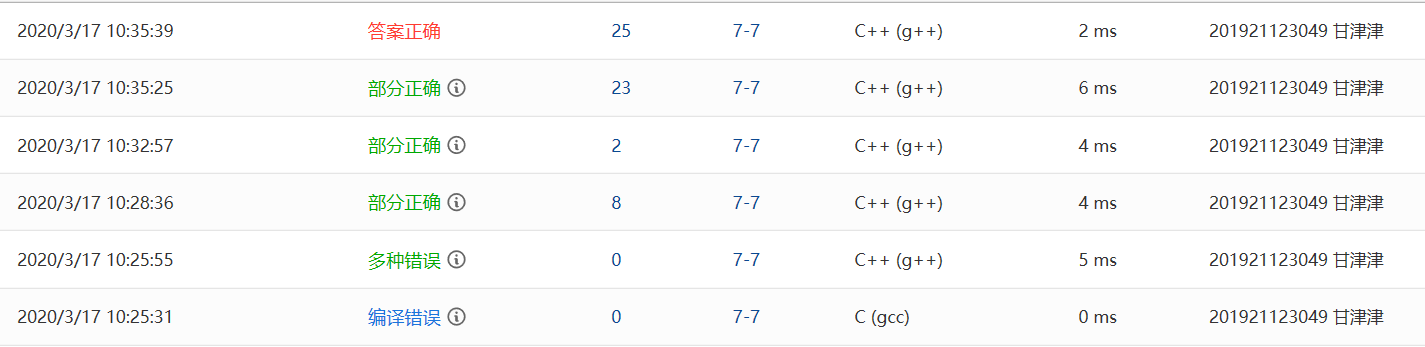• 编译错误：选错了编译器。

• 多种错误：有段错误、格式错误，以下我展开叙述。

• 段错误：定义str[MaxSize]数组时MaxSize 值取得偏小，改为1000即可。

• 格式错误：没看见题干中“但最后一个编号后不能有多余的空格”一句，后将代码改成：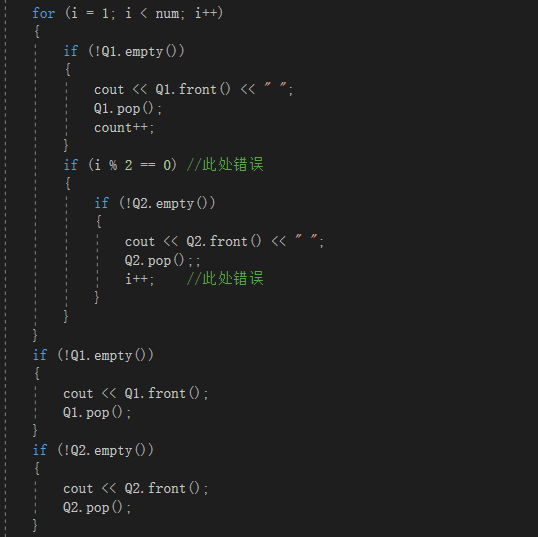• 部分正确：部分测试点显示答案错误，由于在输出Q2数据时也应将i加一，因此i就不能作为判断是否轮到Q2输出的变量，因此另设count作为判断变量，测试点就全过了。

2.2 题目2字符串是否对称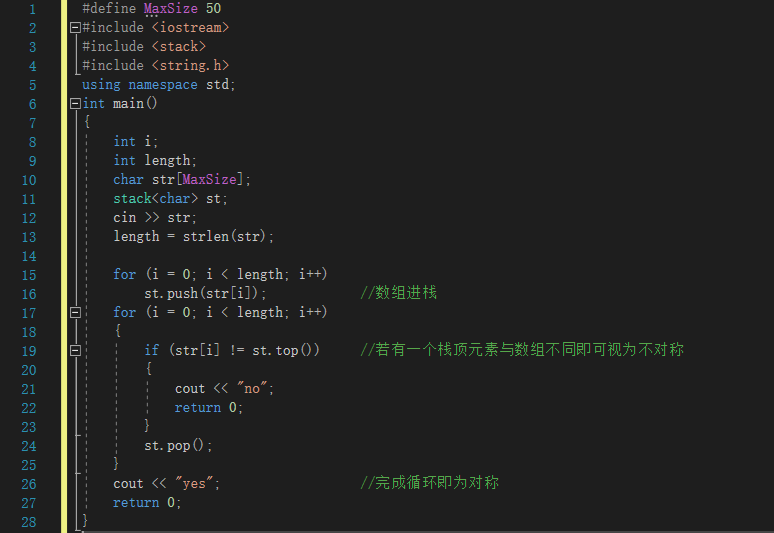## 2.1.2本题PTA提交列表说明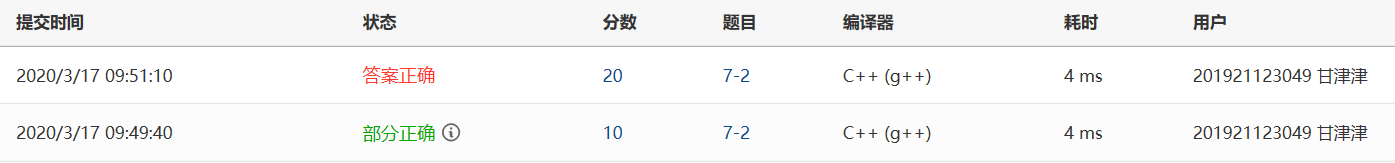• 部分正确：“对称字符串”这一测试点无法通过，经查，为以下错误代码: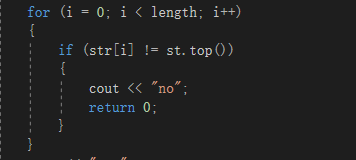# 3.阅读代码

## 3.1 题目及解题代码

• 题干：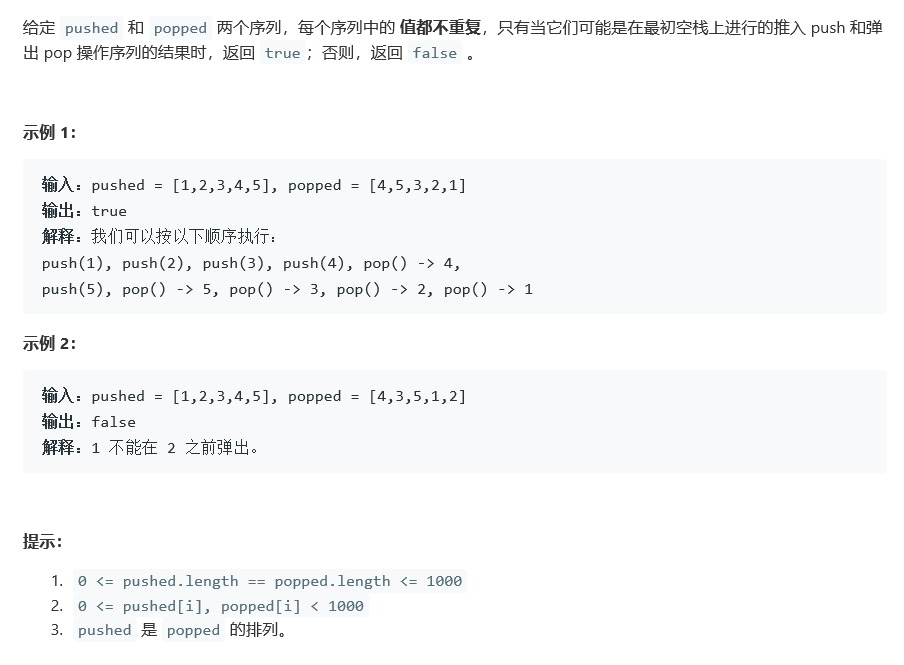• 代码：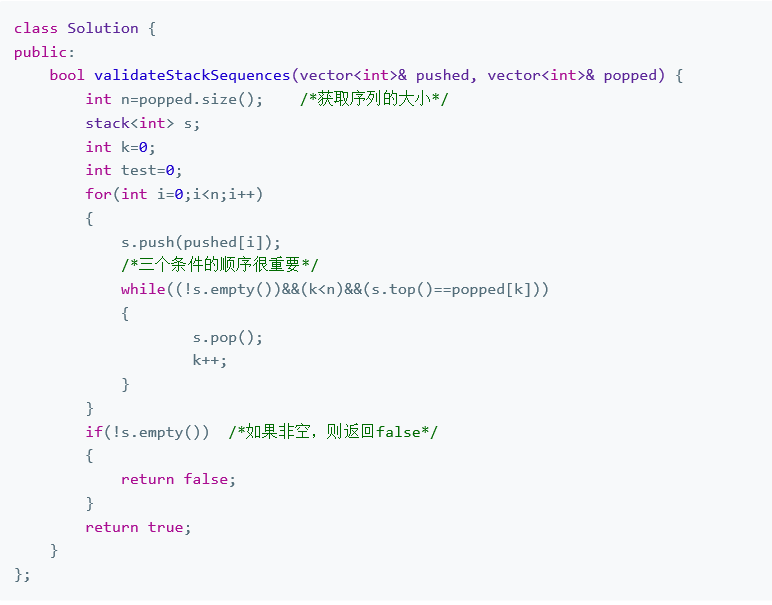### 3.1.1 该题的设计思路

• 思路：1.引入一个栈stack。2.把pushed序列按顺序放到栈stack中，每放一个数据对比栈顶与序列popped中元素是否相同，若相同，则栈stack执行pop操作，移到popped的下一个元素，继续对比栈顶与该元素是否相同。

• 正确案例图解：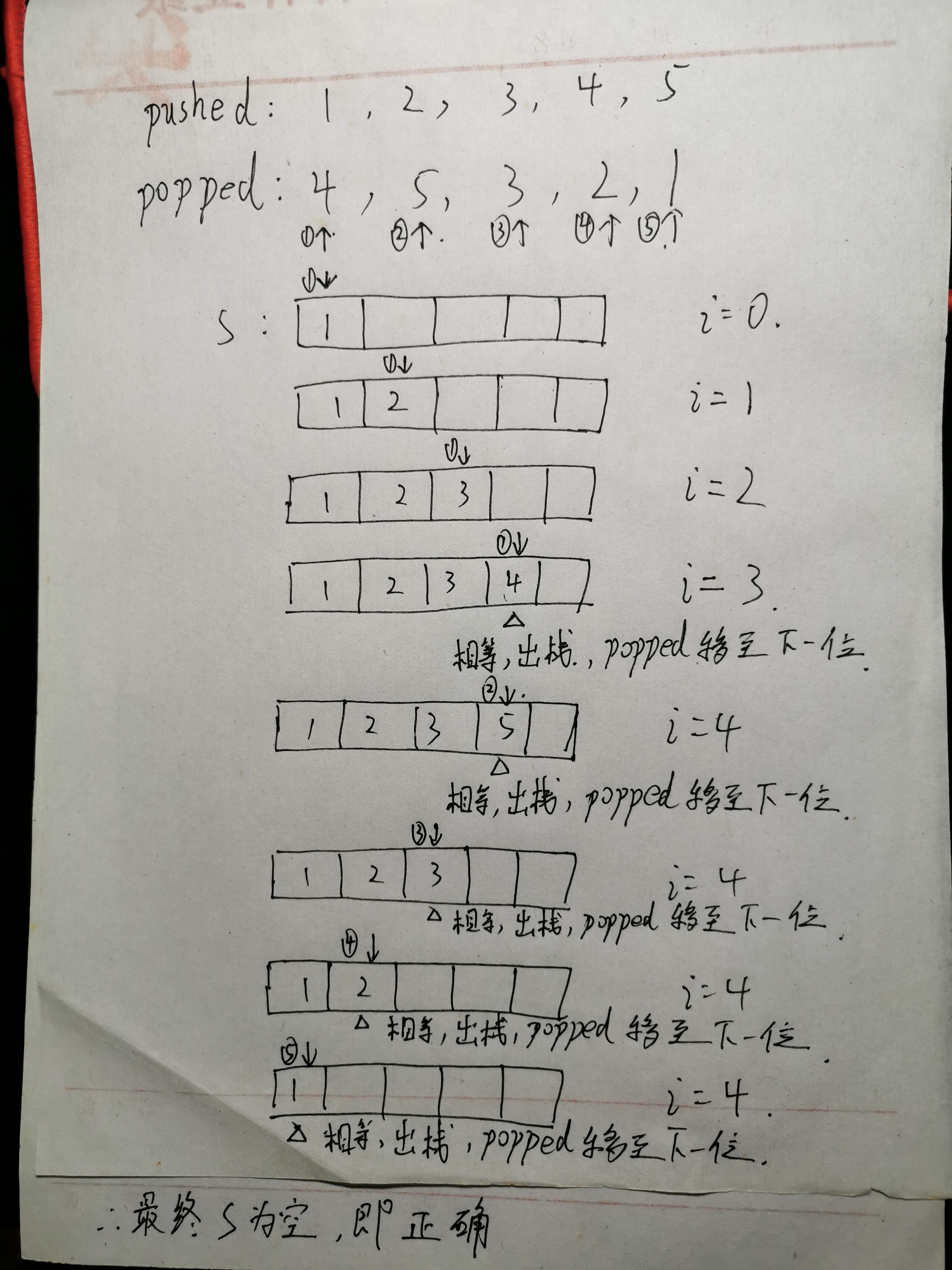• 错误案例图解：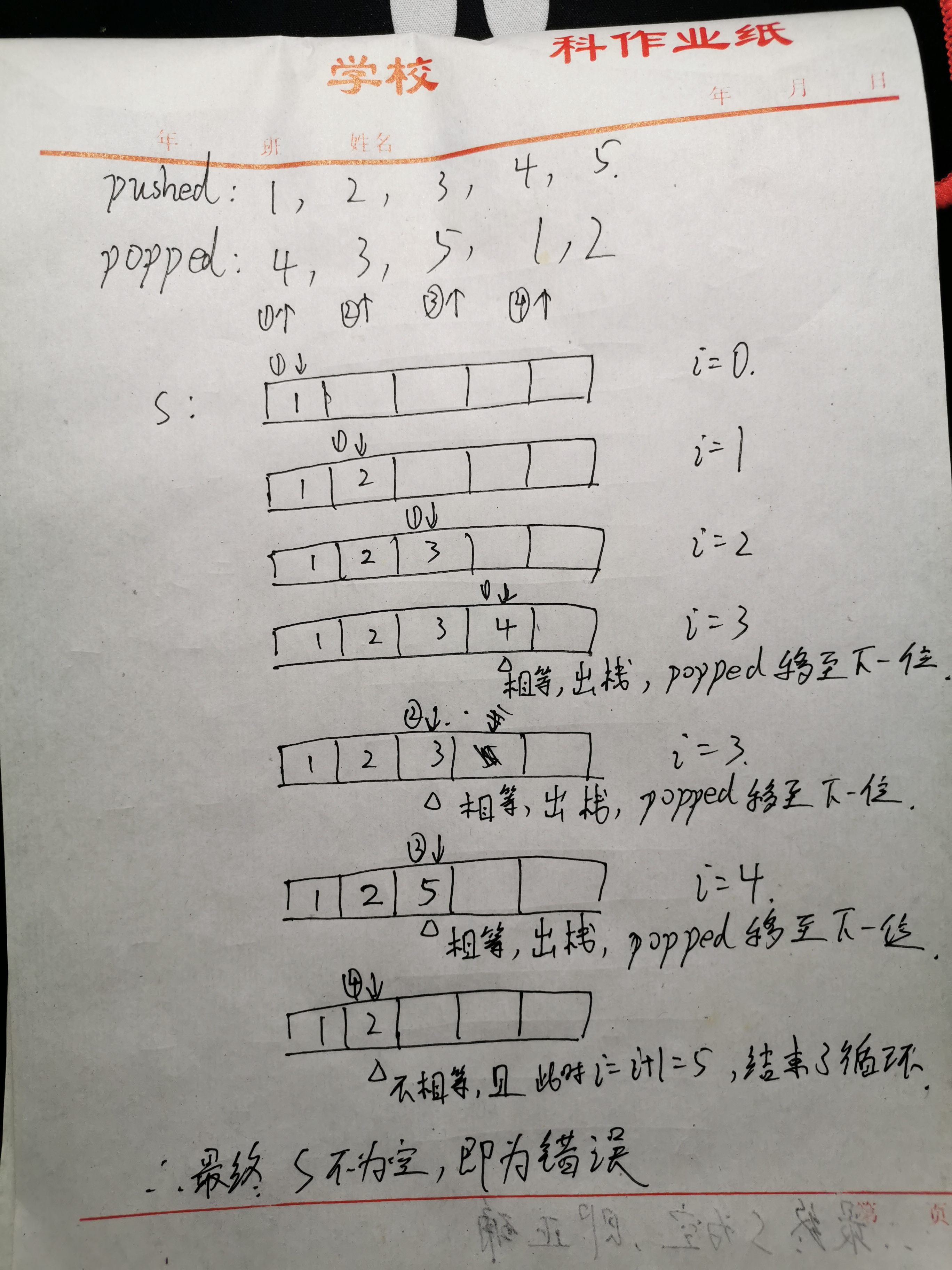• 时间复杂度：以正确的序列为例，假设pushed 和 popped 两个序列的长度为n，则for循环中必定要跑n趟才能将pushed中的所有元素入s栈，而在while循环中也必定需要n趟才能实现s空栈，所以，总次数为2n次，即时间复杂度为O(n)。

• 空间复杂度：O(n)。

### 3.1.2 该题的伪代码

int n=popped.size();    /*获取序列的大小*/
int i；                 //用于for循环
stack<int> s;           //存放pushed元素
int k=0;                //用于统计有几个s栈顶元素与popped首元素相同
for i=0 to i<n
pushed元素依次入s栈
while s非空且k小于n且s栈顶与popped首元素相同
s出栈
k++;
end while
end for
if s非空 then          /*如果非空，则返回false*/
return false;
end if
return true;


### 3.1.3 运行结果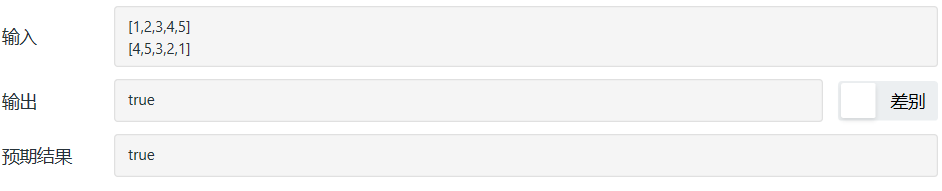### 3.1.4分析该题目解题优势及难点

• 解题优势：思路很棒，利用了栈先进后出的特点仅用两层循环就解决了验证栈的序列，总体代码量也较少，时间及空间复杂度较低。

• 本题难点：给出栈的入栈次序判断栈的出栈次序是否合理本身就是个难点，栈的出栈次序存在着多种组合，编程思路就难稿。

## 3.2 题目及解题代码

• 题目：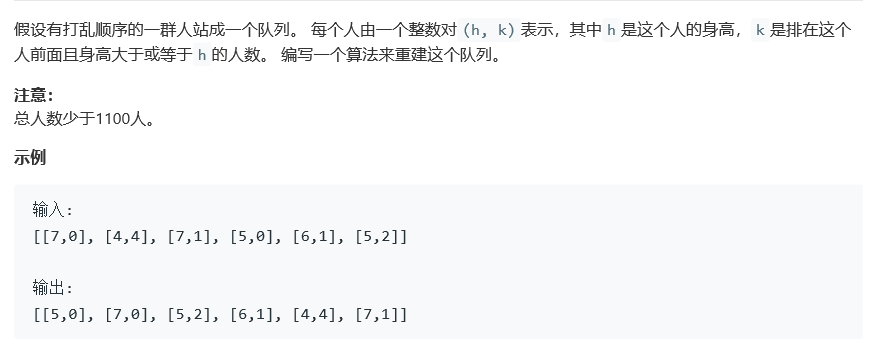• 代码：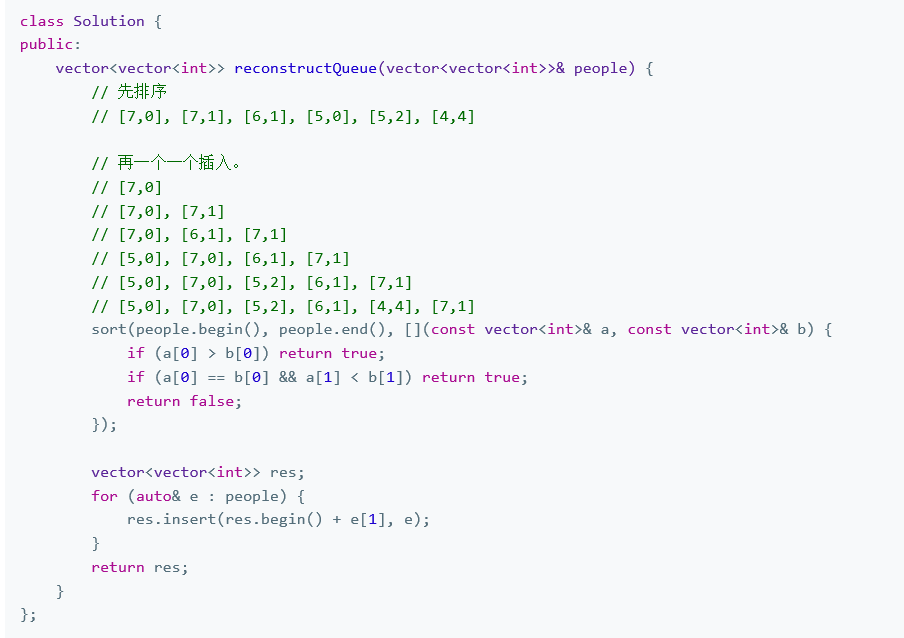### 3.2.1 该题的设计思路

• 思路：

• 图解：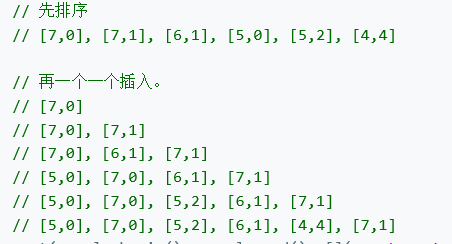• 时间复杂度：两次遍历，一次排序，一次插入，所以时间复杂度为O(n)

• 空间复杂度：O(n)

### 3.2.2 该题的伪代码

将people按照身高降序排序，相同身高需要按k升序排序

for 遍历e
找到位置后插入res
end for



### 3.2.3 运行结果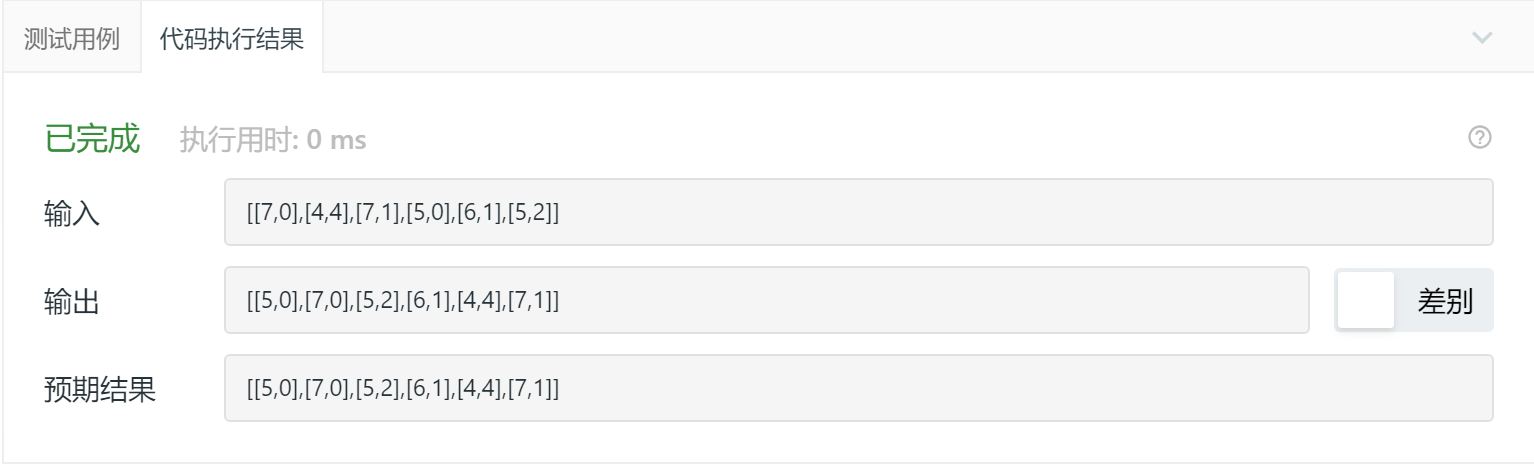### 3.2.4分析该题目解题优势及难点

• 优点：思路很棒 ，代码也很简洁，sort、vector语法运用自如。

• 难点：单按h排序并不难，难在还要考虑k的存在。

posted @ 2020-03-22 20:06  甘津津  阅读(199)  评论(0编辑  收藏  举报